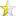• Articles
• C++ class for generate Fibonacci series
Aug 9, 2016 (last update: Aug 9, 2016)

# C++ class for generate Fibonacci series``12345678910111213141516171819202122232425262728293031`` ``````#include using namespace std; class Fibonacci{ public: int a, b, c; void generate(int); }; void Fibonacci::generate(int n){ a = 0; b = 1; cout << a << " " <> n; Fibonacci fibonacci; fibonacci.generate(n); return 0; }``````